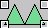JHotDraw Pattern Language

Creating Composite Figures

CompositeFigures are collections of existing figures organised together into a single figure using the Composite design pattern. In JHotDraw this functionality is represented in the abstract CompositeFigure class and its subclass GroupFigure.An example of a composite figure

When creating a custom composite figure there are several issues to consider: selecting which classes are going to be composed, positioning those classes in the composite and finally defining how the composite will react to movement and resize events?

Figures can be added to a composite by calling its add(Figure) method  When adding figures to a composite each figure's position relative to the others has to be defined before composing them together (see example below for details). When figures overlap in a composite It is also important to add the figures in a back to front ordering (i.e. the figure at the back of the composite should be added first).

The GroupFigure class provides a default implementation of composite functionality; it provides support for moving the composite around the drawing but prevents resize behaviour.

• How to create a composite figure (example creates composite figure shown above)

...
TriangleFigure triangle1 = new TriangleFigure(new Point(0,0), new Point(20,20));
TriangleFigure triangle2 = new TriangleFigure(new Point(20,0), new Point(40,20));

GroupFigure group = new GroupFigure();